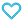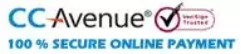# Rank Up Physics JEE Main & Advanced Electricity and Magnetism

425.00

• Paper Book

Discount offer on this book in a bundle, click to view

MTG’s Rank Up Physics for JEE Main & Advanced is a Physics book series comprising 5 books. The series is created by Physics experts to ensure that a JEE (M+A) aspirant can cover the Physics syllabus of JEE exam from all dimensions. The book is an excellent companion for students who wish to learn physics from scratch.
To develop a strong understanding of concepts, the chapters are well explained in a simple language and along with solved intext examples and fundamental questions. The exercises and previous years’ questions in each volume are well equipped with answer keys and explanations so that the student can self-evaluate their understanding of concepts

250 in stock

Qty:Add to WishlistMTG’s Rank Up Physics is an exhaustive book series for the preparation of the Physics syllabus of JEE Main & Advance. The book series includes Five books namely-
• Mechanics Volume 1 (Covers: Basic Mathematics, Unit, Dimensions, and Errors, Vectors, Motion, Projectile Motion, Laws of Motion, Work, Energy and Power, Circular Motion, and Centre Of Mass and Conservation of Momentum)
• Mechanics Volume 2 (Covers: Rotational Motion, Gravitation, Elasticity, Fluid Mechanics, Simple Harmonics)
• Waves And Thermodynamics (Covers: Wave Motion, Superposition of waves, Sound Waves, Thermal Properties and Kinetic Theory, Thermodynamics and Heat Transfer)
• Electricity And Magnetism (Covers: Electrostatics, Capacitors, Electric Current, Magnetic Force, and Magnetic Field, Electromagnetic Induction, and Alternating Current)
• Optics And Modern physics (Covers: Geometrical Optics, Wave Optics, Atomic Physics, Nuclear Physics)
The uniqueness of this series lies in the following fact:
• Complete coverage of syllabus.
• Simplified presentation of complex Physics concepts.
• Numerous solved examples, questions & numerical for each chapter.
• Variety of questions like – single correct answer type, multiple correct answer type, passage-based, matrix match, integer type, etc. to prepare you for both JEE Main & Advanced.
• Previous 2 years’ (2020 & 2021) questions of JEE Main and JEE Advanced with detailed solutions.

Content:
• Unit 1 : Electrostatics
• Electrostatics
• Electric Charge
• Coulomb’s Law
• Principle of Superposition
• Force between Two Point Charges in Vector Form
• Equilibrium of System of Charges
• Electric Field
• Electric Field Lines
• Electrostatic Potential Energy
• Electrostatic Potential
• Relation Between E and V
• Equipotential Surface
• Continuous Charge Distribution
• Electric Dipole
• Electric Field Due to a Dipole
• Potential Due to a Dipole
• Dipole Kept in Uniform External Electric Field
• Electric Flux
• Gauss’s Theorem
• Concept of Solid Angle
• Application of Gauss’s Theorem
• Self Energy
• Properties of Conductor
• Corona Discharge
• Electrostatic Pressure on the Surface of the Charged Conductor
• Energy Density
• Metal Plates : Charge on All the Surfaces
• Separation of Hemispherical Shell
• Electric Field and Potential Due to Induced Charges
• Earthing
• Exercise
• Unit 2 : Capacitors
• Capacitors
• Parallel Plate Capacitor
• Other Types of Capacitors
• Energy Stored in a Capacitor
• System of Capacitors
• Simplifying Circuits
• Parallel Plate Capacitor with Dielectric
• RC Circuits
• Exercise
• Unit 3 : Electric Current
• Electric Current
• Flow of Current in Conductors
• Ohm’s Law
• Resistance
• Emf and Its Sources
• Combination of Resistors
• Kirchhoff’s Law
• Superposition Principle
• Short-circuit
• Wheatstone’s Bridge
• Equipotential Points
• Symmetry of Path
• Electrical Bulb
• Electrical Instruments
• Exercise
• Unit 4 : Magnetic Force and Magnetic Field
• Introduction
• Motion of Charged Particle in a Uniform Magnetic Field
• Path of a Charged Particle in Combined Electric and Magnetic Field
• Magnetic Force on a Current Carrying Conductor
• Torque on a Current Loop
• Magnetic Dipole
• Potential Energy of a Magnetic Dipole in a Magnetic Field
• Ratio of Magnetic Moment to the Angular Momentum of the Electron
• Applications of Moving Charged Particle in a Magnetic Field
• Magnetic Field Due to Moving Charges
• Force between Two Infinite Parallel Current Carrying Wire
• Magnetic Field on the Axis of the Circular Current Carrying Ring
• Magnetic Field Due to Solenoid
• Ampere’s Law
• Bar Magnets
• Force, Torque and Potential Energy of a Bar Magnet Kept in a Uniform Magnetic Field
• Moving Coil Galvanometer
• Magnetic Materials
• Magnetic Hysteresis
• Terrestrial Magnetism
• Exercise
• Unit 5 : Electromagnetic Induction
• Magnetic Flux
• Lenz’s Law
• Motional Emf
• Potential Difference Across the Ends of Rotating Rod
• Velocity of a Rod (Slider) as a Function of Time
• Self Induction
• Energy Stored in an Inductor
• LR Circuits
• Mutual Inductance
• Relation between Self and Mutual Induction
• Combination of Inductors
• Induced Electric Field
• LC Oscillations
• Transformers
• Exercise
• Unit 6 : Alternating Current
• Alternating Current
• AC Voltage Generation
• Hot Wire Instruments
• Types of AC Circuit
• Rejector Circuit
• Power in AC Circuits
• Choke Coil
• Exercise